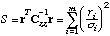# Manual Page for Command >>> LEAST-SQUARES

Syntax
>>> LEAST-SQUARES

Parent Command
>> OPTION

Subcommand
-

Description
This command selects least-squares optimization, i.e., the objective function to be minimized is the sum of the squared weighted residuals.Minimizing the squared weighted residuals leads to a maximum-likelihood estimate if the errors are normally distributed with zero mean and covariance matrix Czz:Least-squares estimation is the default. If outliers are more prominent than described by the tail of the normal distribution, one may want to use one of the robust estimators to reduce the weight of outliers or even eliminate them (see commands >>> ANDREW, >>> CAUCHY, or >>> QUADRATIC-LINEAR). The least-squares objective function is a quadratic function if the residuals depend linearly on the parameters; it is nearly-quadratic for a non-linear model. The Levenberg-Marquardt algorithm is best suited to minimize the non-linear least-squares objective function.

Example
> COMPUTATION
>> OPTION
>>> use LEAST-SQUARES
<<<
<<